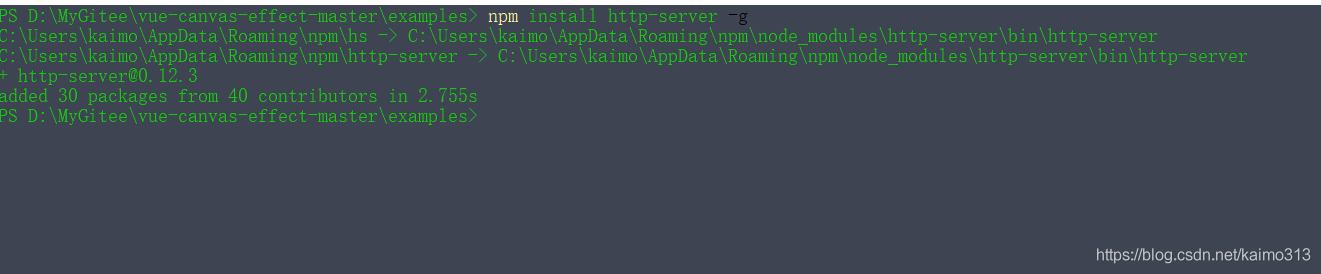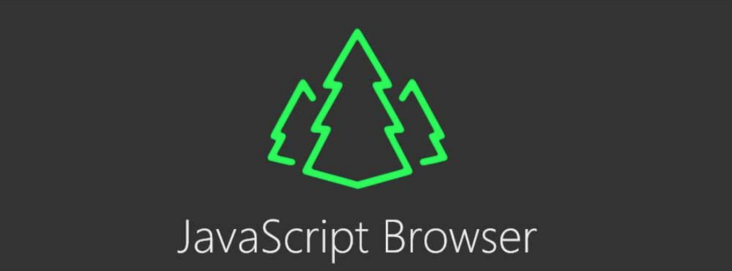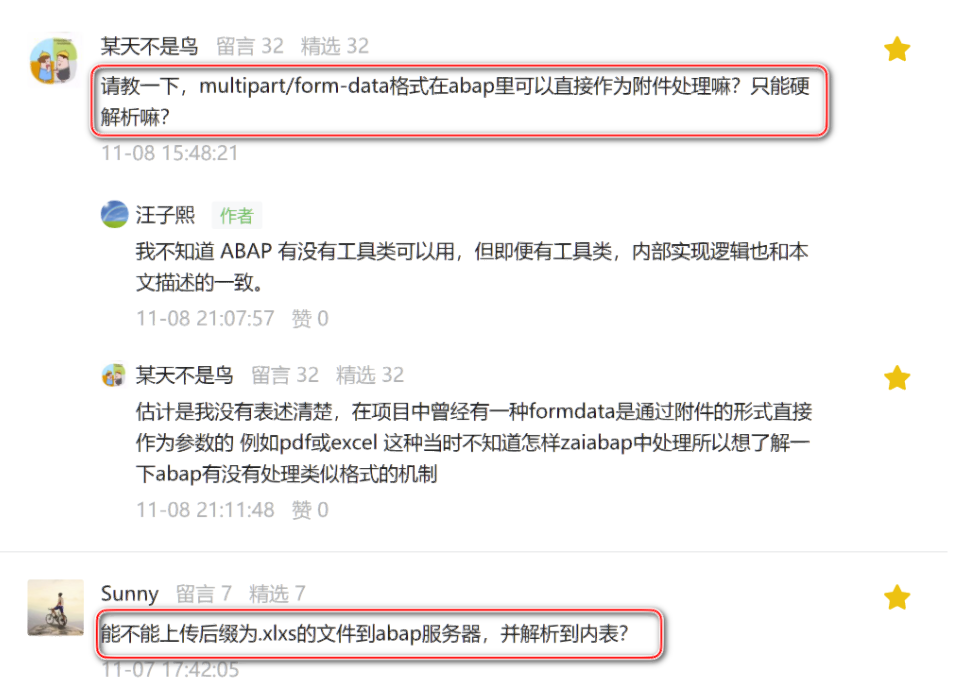# javascript小技巧：同步服务器时间、同步倒计时

+关注继续查看1 2 3 4 5 6 7 8 9 10 11 12 13 14 15 16 17 18 19 20 21 22 23 24 25 26 27 28 29 30 31 32 33 34 35 36 37 38 39 40 <span id="timebox">11:21:55 //第一次将服务器时间显示在这里 <script type="text/javascript">     $(function () {  var oTime =$("#timebox");         var ts = oTime.text().split(":", 3);         var tnums = [parseInt(ts), parseInt(ts), parseInt(ts)];         setInterval(function () {             tnums = getNextTimeNumber(tnums, tnums, tnums);             showNewTime(tnums, tnums, tnums);         }, 1000);         function showNewTime(h, m, s) {             var timeStr = ("0" + h.toString()).substr(-2) + ":"                             + ("0" + m.toString()).substr(-2) + ":"                              + ("0" + s.toString()).substr(-2);             oTime.text(timeStr);         }         function getNextTimeNumber(h, m, s) {             if (++s == 60) {                 s = 0;             }             if (s == 0) {                 if (++m == 60) {                     m = 0;                 }             }             if (m == 0) {                 if (++h == 24) {                     h = 0;                 }             }             return [h, m, s];         }     }); 

 1 2 3 4 5 6 7 8 9 10 11 12 13 14 15 16 17 18 19 20 21 22 23 24 25 26 27 28 29 30 31 32 33 34 35 36 37 38 39 40 41 42 43 44 45 46 47 48 49 50 51 52 53 54 55 56 57 58 59 60 61 62  <html> <head>     <title>同步倒计时     <script type="text/javascript" src="jquery-1.4.4.min.js">  <body>     <span id="timebox">1天00时00分12秒      <script type="text/javascript">         $(function () {  var tid = setInterval(function () {  var oTimebox =$("#timebox");                 var syTime = oTimebox.text();                 var totalSec = getTotalSecond(syTime) - 1;                 if (totalSec >= 0) {                     oTimebox.text(getNewSyTime(totalSec));                 } else {                     clearInterval(tid);                 }             }, 1000);             //根据剩余时间字符串计算出总秒数             function getTotalSecond(timestr) {                 var reg = /\d+/g;                 var timenums = new Array();                 while ((r = reg.exec(timestr)) != null) {                     timenums.push(parseInt(r));                 }                 var second = 0, i = 0;                 if (timenums.length == 4) {                     second += timenums * 24 * 3600;                     i = 1;                 }                 second += timenums[i] * 3600 + timenums[++i] * 60 + timenums[++i];                 return second;             }             //根据剩余秒数生成时间格式             function getNewSyTime(sec) {                 var s = sec % 60;                 sec = (sec - s) / 60; //min                 var m = sec % 60;                 sec = (sec - m) / 60; //hour                 var h = sec % 24;                 var d = (sec - h) / 24;//day                 var syTimeStr = "";                 if (d > 0) {                     syTimeStr += d.toString() + "天";                 }                 syTimeStr += ("0" + h.toString()).substr(-2) + "时"                             + ("0" + m.toString()).substr(-2) + "分"                             + ("0" + s.toString()).substr(-2) + "秒";                 return syTimeStr;             }         });http-server：一个JavaScript服务器
http-server：一个JavaScript服务器
8 0JS获取服务器日期，自动切换网页信息
JS获取服务器日期，自动切换网页信息
47 0Node.js 创始人：Javascript 容器是简化服务器抽象的“先驱”践行者
Node.js 创始人：Javascript 容器是简化服务器抽象的“先驱”践行者
45 0Node.js 创始人：Javascript 容器是简化服务器抽象的“先驱”践行者
Node.js 创始人：Javascript 容器是简化服务器抽象的“先驱”践行者
71 0JavaScript 服务器推送技术之 WebSocket

92 0191 070 0123 0

JavaScript 自学手册文档教程323165

JavaScript入门与实战19215521381989120326

Linux Web服务器Nginx搭建与配置136615

Javascript中的函数

Javascript异步编程

JS零基础入门教程（上册）